## Bass Boost Calculator

These calculators are based on the formulas derived in this Head-Fi thread.

### Definitions

ƒc — The frequency at which the boost is +3 dB; the corner frequency of the filter. Below this frequency, the PPA bass boost increases by 6 dB each time you divide the frequency in half, up to the point where the shelving action kicks in.

ƒs — The frequency at which the boost curve is 3 dB below the maximum boost value; the shelving frequency of the filter.

ƒo — Frequency where the gain is half the total boost. Used when boost is 6 dB or less, since ƒc and ƒs cross each other at this point.

### I Know What Resistor Values I Will Use, What C7 Will Give Me the ƒc I Want?

This calculator will help you pick a C7 value given the ƒc and the R3, R4 and R7 values. The answer will not be rounded off to a standard value, since different capacitor lines have different standard values.

You may use power notation for the resistors: 120K, 1.0M, etc. The power suffix can be uppercase or lowercase.Given: R3: Ω R4: Ω R7: Ω ƒc: Hz This calculator requires JavaScript.

The formula for this is: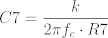where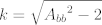and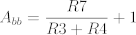### Given All the Component Values, What Filter Characteristics Will I Get?Given: R3: Ω R4: Ω R7: Ω C7: µF This calculator requires JavaScript.

The formulæ for this are: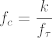,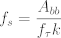and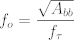where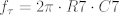This space intentionally left blank. :)Updated Sun Jan 18 2015 04:24 MST Go back to The PPA Project Go to my home page